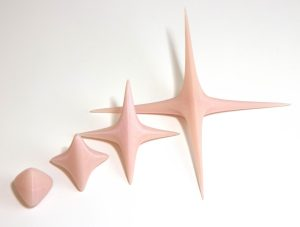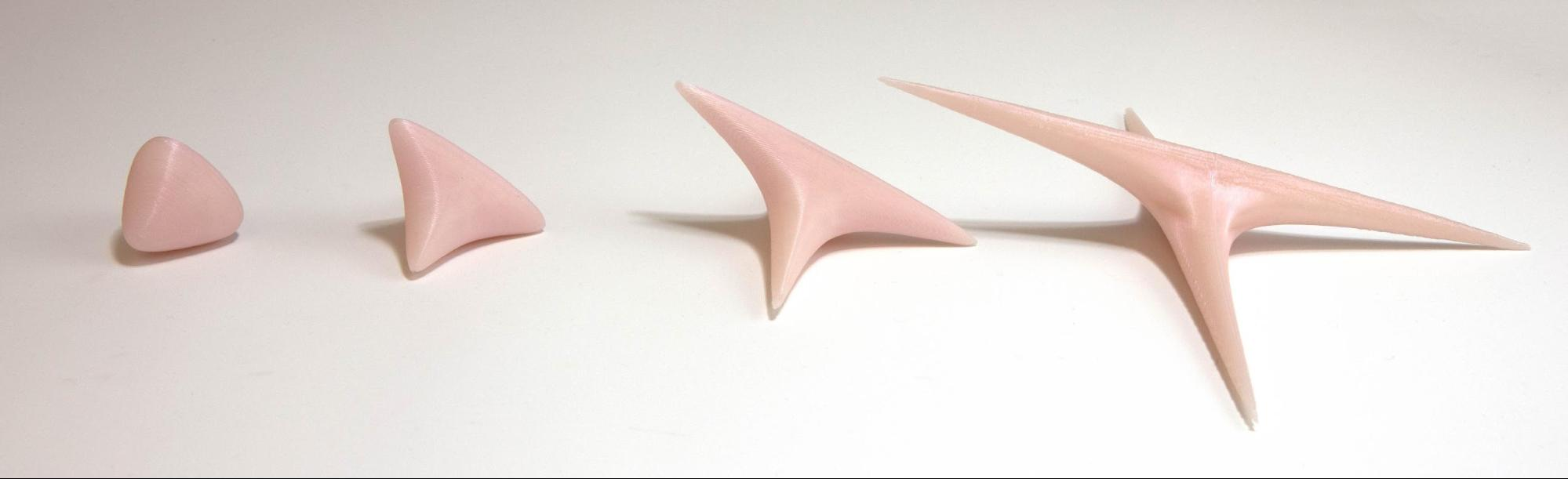# Balls in Nil and Sol

Date started: October 2019

###Abstract

In his work on 3-dimensional manifold Thurston proved that there are exactly 8 “geometries” that serve as model for any compact three dimensional manifold. Some of them are familiar to mathematicians, e.g. euclidean, spherical or hyperbolic geometries. Other are rather more exotic.

The Nil and the Sol geometries correspond to the suspension of a torus by a Dehn twist and an Anosov map respectively. The underlying topological space of these two geometries is simply$\mathbf R^3$. However the distance between two points is not the usual one. A natural question is “What is the shape of balls in these geometries?”

The goal of this project is to produce 3D printed models of balls in the Nil and Sol geometries.

This project was motivated by Rich Schwartz lectures at the ICERM during Fall 2019.

### Media